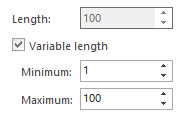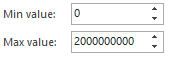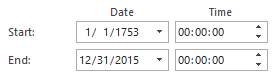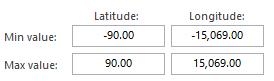# How to generate randomized test data in ApexSQL Generate

Applies to
ApexSQL Generate

Summary
This article explains how to generate randomized data in ApexSQL Generate by using the Random generator. Also, it shows how it works for basic SQL data types.

Description

The Random generator creates a sequence of randomized values from the start up to the end value. The generated sequence of values is created in the pseudo-random (seeded random) manner, which means that the sequence depends on the value of Seed. More info on the Seed and the way it affects the generated sequence can be found on this link.

The Random generator has 3 options which are present for any given SQL type:

1. Seed value – determines the order of values in the generated sequence
2. Unique – filters out all repeating values from the generated sequence, leaving only one instance of each value. If a column has a unique constraint assigned to it, this option is automatically checked
3. Allow null – inserts the NULL values into the generated sequence. The number of NULL values is determined as the percentage of requested row number for the table containing the selected column. The percentage value is assigned through the % of null option.The Random generator can be set for a column of any SQL type. The options and functionality of the generator depend on the SQL type of the column for which it is set. There are 6 different types of random generator:

1. Character – generates a series of random characters
2. Numeric – generates random numeric values in a given range
3. Datetime – generates random dates in a given range
4. Image – generates random binary values representing the SQL image type with dimensions from the given range
5. Spatial – generates random values for geography and geometry SQL types from the given range
6. Universal – used for generating random values for all other SQL types (xml, GUID, hierarchyId, etc.)

Character random generatorThis type of random generator creates a series of random characters of a specific length.
The Character random generator is used for any character (char, nvarchar, text) and binary SQL type.

The number of characters in one row is set through the Length value. The maximum number which can be set in the Length field depends on the definition of the given SQL type. For instance, if a column is of a nvarchar(25) type, the maximum length of the generated string for each row cannot go above 25. For nvarchar(100) type, maximum length is 100 (as in the picture above).

The number of characters for each row can be set to a static value (i.e. 100 characters) or to a random number in a given range from Minimum up to Maximum length. To switch between static and variable length of the string generated for each row, Variable length option is used.
Minimum value cannot go below 1 and Maximum value cannot go above the value defined in the SQL type.

Numeric random generatorThis type of generator creates a single random number for each row from the given range of values. It is used for all numeric (int, float, decimal, smallint, etc.) SQL types.

The lowest and highest value which can be set in the Min and Max value fields depend on the SQL type of the column for which this generator is chosen. For instance, if the selected column is of an int type, the minimum value is -2,147,483,648 and maximum value is 2,147,483,647, according to the definition of the int SQL type. The same rule is applied to all other numeric SQL types.

The default values are from 0 to a rounded number closest to the maximum for that type. In the example of the int type, default maximum value is 2000000000 (as in the image above).

Datetime random generatorThis type of random generator creates a single random datetime value for each row, from the given range of values. It is used for all variations of datetime SQL type (datetime, date, time, and datetimeoffset). For date SQL type, only Start date and End date fields are shown, and for time SQL type, only Start and End time fields are shown.

There is no limitation for the start and end values. Default values for date are from 1/1/1753 (commonly used as a minimum value for SQL datetime type) up to the current date (when the project is created).

Spatial random generatorThis type of random generator creates a random geography/geometry value for each row, from a given range. The generated value is a point in space, the generator is not able to create a complete polygon.

Default values are corresponding to the minimum and maximum values for these SQL types, and the generator allows setting the values only in that range, in order to prevent the entries which conflict with the logic of the underlying SQL types.

Universal random generator

This type of generator is used for all other SQL types (xml, GUID, hierarchyId, sqlvariant, etc.) and does not have any specific settings other that the 3 above mentioned standard settings for the random generator (seed, unique, allow null).

Calculation of default values based on the CHECK constraints

The random generator has the ability to recognize the simple CHECK constraints set for the column for which the generator is chosen. In this case, standard default values are overridden with the values set in the constraint. This provides additional automatic recognition of proper settings for the selected column and is applicable to any SQL type, as long as the ApexSQL Generate recognizes the constraint properly. The most easily recognized constraints are the ones which define the range of allowed values. For instance, if the column named Column1 is of an int type, and the constraint states:

`Column1 > 10 AND Column1 < 100`

the generator will set its settings automatically to reflect the range from 10 up to 100.

Top FAQ’s

• Q: For which data types can I use the Random generator?

A: Random generator can be used for any SQL data type, except server assigned (identity, timestamp, computed, primary key)

• Q: How do I repeat a previously generated sequence in the same exact order?

A: The generated sequence depends on the assigned range of possible values and the Seed value. More info on the Seed value and how it affects the random generator’s generated sequence can be found on this link

• Q: What can I use Random generator for?

A: This type of generator is one of the most versatile generators and has the ability to recognize the CHECK constraints of the column for which it is chosen. This allows the automatic generation of correct data, so it can be used for quick and easy generation of test data in few clicks upon the loading of the database. It can also be used for generating data for testing real-life databases’ behavior when storing large amount of data without predictable pattern.

• Q: What does the Unique option mean and how does it work?

A: The Unique option allows creation of sequence which contains only one instance of each value from the given range. If the number of possible unique values from the given range is lower than the requested number of rows for the table containing the selected column, the number of generated rows for each column in the table is reduced to the number of possible unique values.

For example, if a column of an int type has the random generator assigned to it, with the range set from 0 to 3, only 4 values are generated (0, 1, 2, 3), in a random order depending on the Seed value.

Note that when Unique and Allow null options are checked with the percentage of null values greater than 0, the generated sequence will contain only one NULL value.

• Q: Does Random generator recognizes the check constraints I have set for my column?

A: Yes, the Random generator has the ability to recognize the CHECK constraints set for the selected column and to adjust its settings accordingly. The constraints which limit the range of allowed values are the ones most easily recognized (e.g. value > 1 AND value < 10). The recognition is done automatically and can be overridden by changing the generator’s settings.